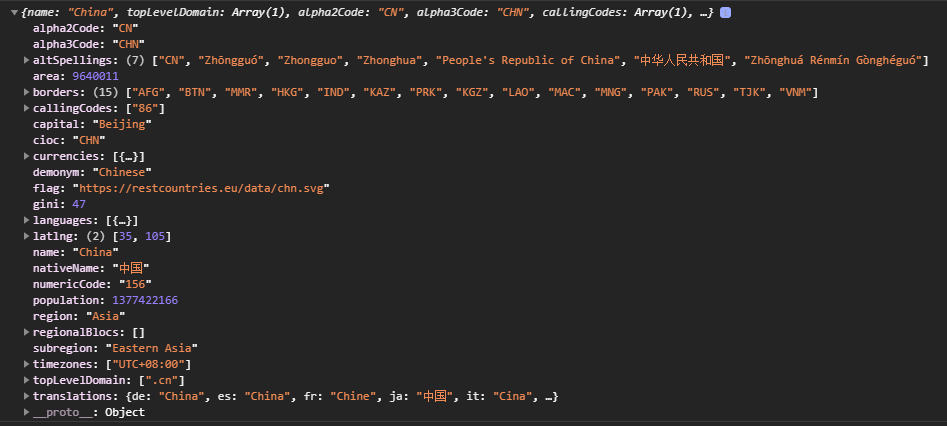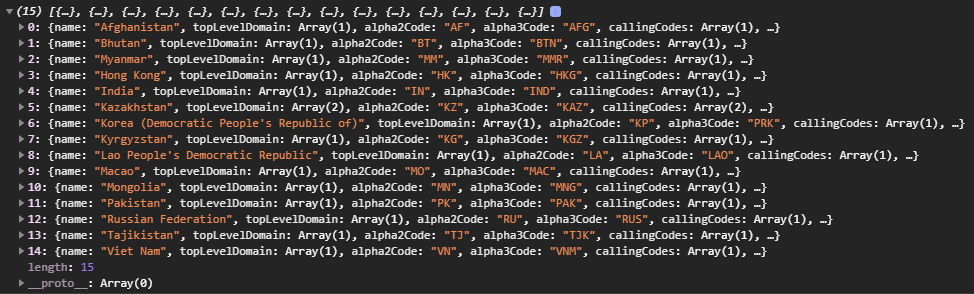20分钟带你掌握JS Promise和Async-Await

• 示例1：用生日解释Promise的基础知识
• 示例2：一个猜数字的游戏
• 示例3：从Web API中获取国家信息
• 示例4：从Web API中获取一个国家的周边国家列表

# 示例1：用生日解释Promise基础知识

Promise执行时分三个状态：pending（执行中）、fulfilled（成功）、rejected（失败）。

``````new Promise(function(resolve, reject) {
if (/* 异步操作成功 */) {
} else {
}
})``````

``````promise
.then((result) => {
//promise被接收或拒绝继续执行的情况
})
.catch((error) => {
//promise被拒绝的情况
})
.finally (() => {
//promise完成时，无论如何都会执行的情况
})``````

``````const onMyBirthday = (isKayoSick) => {
return new Promise((resolve, reject) => {
setTimeout(() => {
if (!isKayoSick) {
resolve(2);
} else {
}
}, 2000);
});
};``````

• 如果Kayo没有生病，那么我们就以2为参数执行resolve函数

``````onMyBirthday(false)
.then((result) => {
console.log(`I have \${result} cakes`); // 控制台打印"I have 2 cakes"
})
.catch((error) => {
console.log(error); // 不执行
})
.finally(() => {
console.log("Party"); // 控制台打印"Party"
});``````

``````onMyBirthday(true)
.then((result) => {
console.log(`I have \${result} cakes`); // 不执行
})
.catch((error) => {
console.log(error); // 控制台打印"我很难过"
})
.finally(() => {
console.log("Party"); // 控制台打印"Party"
});``````

# 示例2：一个猜数字的游戏

• 用户可以输入任意数字
• 系统从1到6中随机生成一个数字
• 如果用户输入数字等于系统随机数，则给用户2分
• 如果用户输入数字与系统随机数相差1，给用户1分，否则，给用户0分
• 用户想玩多久就玩多久

``````const enterNumber = () => {
return new Promise((resolve, reject) => {
// 从这开始编码
});
};``````

``````const enterNumber = () => {
return new Promise((resolve, reject) => {
const userNumber = Number(window.prompt("Enter a number (1 - 6):")); // 向用户索要一个数字
const randomNumber = Math.floor(Math.random() * 6 + 1); // 选择一个从1到6的随机数
});
};``````

``````const enterNumber = () => {
return new Promise((resolve, reject) => {
const userNumber = Number(window.prompt("Enter a number (1 - 6):")); // 向用户索要一个数字
const randomNumber = Math.floor(Math.random() * 6 + 1); //选择一个从1到6的随机数

if (isNaN(userNumber)) {
reject(new Error("Wrong Input Type")); // 当用户输入的值非数字，抛出异常并调用reject函数
}
});
};``````

``````return new Promise((resolve, reject) => {
const userNumber = Number(window.prompt("Enter a number (1 - 6):")); // 向用户索要一个数字
const randomNumber = Math.floor(Math.random() * 6 + 1); // 选择一个从1到6的随机数

if (isNaN(userNumber)) {
reject(new Error("Wrong Input Type")); // 当用户输入的值非数字，抛出异常并调用reject函数
}

if (userNumber === randomNumber) {
// 如果相等，我们给用户2分
resolve({
points: 2,
randomNumber,
});
} else if (
userNumber === randomNumber - 1 ||
userNumber === randomNumber + 1
) {
// 如果userNumber与randomNumber相差1，那么我们给用户1分
resolve({
points: 1,
randomNumber,
});
} else {
// 否则用户得0分
resolve({
points: 0,
randomNumber,
});
}
});``````

``````const continueGame = () => {
return new Promise((resolve) => {
if (window.confirm("Do you want to continue?")) { // 向用户询问是否要继续游戏
resolve(true);
} else {
resolve(false);
}
});
};``````

``````const handleGuess = () => {
enterNumber() // 返回一个Promise对象
.then((result) => {
alert(`Dice: \${result.randomNumber}: you got \${result.points} points`); // 当resolve运行时，我们得到用户得分和随机数

// 向用户询问是否要继续游戏
continueGame().then((result) => {
if (result) {
handleGuess(); // If yes, 游戏继续
} else {
alert("Game ends"); // If no, 弹出游戏结束框
}
});
})
};

handleGuess(); // 执行handleGuess 函数``````

``````const handleGuess = async () => {
try {
const result = await enterNumber(); // 代替then方法，我们只需将await放在promise前，就可以直接获得结果

alert(`Dice: \${result.randomNumber}: you got \${result.points} points`);

const isContinuing = await continueGame();

if (isContinuing) {
handleGuess();
} else {
}
} catch (error) { // catch 方法可以由try, catch函数来替代
}
};``````

• 和then函数不同，我们只需将await关键字放在Promise前，就可以直接获得结果。
• 我们可以使用try, catch语法来代替promise中的catch方法。

``````const enterNumber = () => {
return new Promise((resolve, reject) => {
const userNumber = Number(window.prompt("Enter a number (1 - 6):")); // 向用户索要一个数字
const randomNumber = Math.floor(Math.random() * 6 + 1); // 系统随机选取一个1-6的数字

if (isNaN(userNumber)) {
reject(new Error("Wrong Input Type")); // 如果用户输入非数字抛出错误
}

if (userNumber === randomNumber) { // 如果用户猜数字正确，给用户2分
resolve({
points: 2,
randomNumber,
});
} else if (
userNumber === randomNumber - 1 ||
userNumber === randomNumber + 1
) { // 如果userNumber与randomNumber相差1，那么我们给用户1分
resolve({
points: 1,
randomNumber,
});
} else { // 不正确，得0分
resolve({
points: 0,
randomNumber,
});
}
});
};

const continueGame = () => {
return new Promise((resolve) => {
if (window.confirm("Do you want to continue?")) { // 向用户询问是否要继续游戏
resolve(true);
} else {
resolve(false);
}
});
};

const handleGuess = async () => {
try {
const result = await enterNumber(); // await替代了then函数

alert(`Dice: \${result.randomNumber}: you got \${result.points} points`);

const isContinuing = await continueGame();

if (isContinuing) {
handleGuess();
} else {
}
} catch (error) { // catch 方法可以由try, catch函数来替代
}
};

handleGuess(); // 执行handleGuess 函数
``````

# 示例3：从Web API中获取国家信息

https://restcountries.eu/rest/v2/alpha/cn，你会看到JSON格式的国家数据。

``````const fetchData = async () => {
const res = await fetch("https://restcountries.eu/rest/v2/alpha/cn"); // fetch() returns a promise, so we need to wait for it

const country = await res.json(); // res is now only an HTTP response, so we need to call res.json()

console.log(country); // China's data will be logged to the dev console
};

fetchData();``````

# 示例4：从Web API中获取一个国家的周边国家列表

``````const fetchCountry = async (alpha3Code) => {
try {
const res = await fetch(
`https://restcountries.eu/rest/v2/alpha/\${alpha3Code}`
);

const data = await res.json();

return data;
} catch (error) {
console.log(error);
}
};``````

``````const fetchCountryAndNeighbors = async () => {
const china= await fetchCountry("cn");

console.log(china);
};

fetchCountryAndNeighbors();````````````const neighbors =
china.borders.map((border) => fetchCountry(border));
``````

neighbors是一个Promise对象的数组。

``````const fetchCountryAndNeigbors = async () => {
const china = await fetchCountry("cn");

const neighbors = await Promise.all(
china.borders.map((border) => fetchCountry(border))
);

console.log(neighbors);
};

fetchCountryAndNeigbors();````````````const fetchCountry = async (alpha3Code) => {
try {
const res = await fetch(
`https://restcountries.eu/rest/v2/alpha/\${alpha3Code}`
);

const data = await res.json();

return data;
} catch (error) {
console.log(error);
}
};

const fetchCountryAndNeigbors = async () => {
const china = await fetchCountry("cn");

const neighbors = await Promise.all(
china.borders.map((border) => fetchCountry(border))
);

console.log(neighbors);
};

fetchCountryAndNeigbors();``````

# 总结